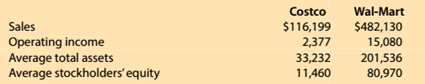Chapter 15, Problem 15.5.3MBA

Chapter
Section
Textbook Problem

Financial leverageCostco Wholesale Corporation (COST) and Wel-Mart Stroes Inc. (WMT)reported the following data (in milllions) for a recent year:Compute the profit margin, asset turnover, and financial leverage metrics. Round profitmargin, asset turnover, and financial leverage to two decimal places.Round return onstockholders’ equity to one decimal place.

To determine

Concept Introduction:

DuPont analysis is an Analysis to examine the Return of Equity (ROE) of a company by various means which includes Profit margin, Asset turnover and financial leverage.

To Calculate:

Profit margin, Assets turnover, Financial Leverage, and Return on Investment

Explanation

Profit margin, Assets turnover, Financial Leverage, and Return on Investment are calculated as follows:

 Costco Wal-Mart Operating Income (A) \$ 2,377 \$ 15,080 Sales (B) \$ 116,199 \$ 482,130 Profit Margin (C) = A/B = 2.05% 3.13% Sales (D) \$ 116,199 \$ 482,130 Average Total Assets (E) \$ 33,232 \$ 201,536 Asset Turnover Ratio (F)= (D/E) = 3...

Still sussing out bartleby?

Check out a sample textbook solution.

See a sample solution

The Solution to Your Study Problems

Bartleby provides explanations to thousands of textbook problems written by our experts, many with advanced degrees!

Get Started